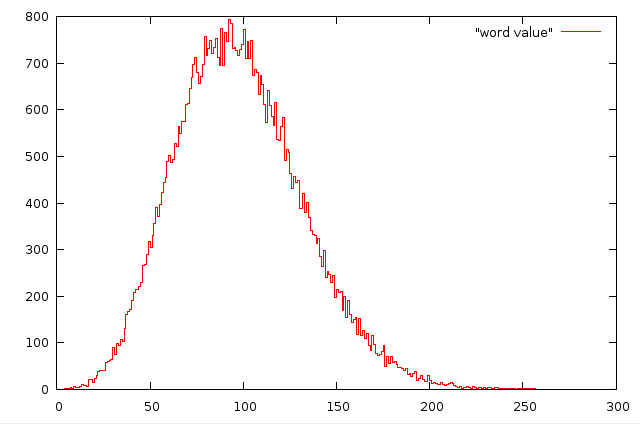#One-Dollar Words Answers

Math Lair Home > Topics > One-Dollar Words Answers

Here are the answers to the problems posed on the One Dollar Words Problems page:

1. The best way to solve this one is to find which number less than 10 has the largest \$1 word value. This happens to be seven. From there, it's not too difficult to find the largest number under 1,000, which is 777.
2. They are 30 and 51.
3. "Three dollars and sixty-six cents" has a \$1 word value of \$3.66.
4. "Two hundred and fifty one" has a \$1 word value of 251¢. The other solution is 259.
5. 29, 25, 38, 66, and 47 all have a \$1 word value of \$1.49.

Here are some thoughts about some of the questions in the "Further investigation" section:

As regards the question "For which value are there the most words?", it shouldn't be too difficult to write a computer program to figure out the answer if you have a dictionary file. I tried this with the Linux dictionary file, ignoring proper nouns, hyphenated words, words with apostrophes, etc., and got the following result:Your results might vary depending on what file you're using, but what I found was that the peak of the graph occurs just before the \$1.00 mark (to be precise, it occurs at 92¢). It would be interesting to try to determine what statistical distribution best fits this distribution.

Note that the numbers on this graph vary from the numbers of words listed on the one-dollar words and \$1.10 words pages; this is because I've ignored proper nouns and used only a single data source.